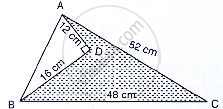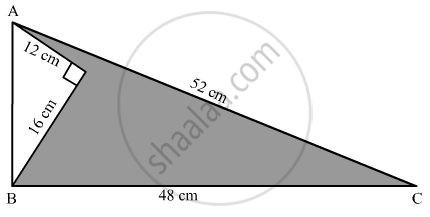# Find the Area of the Shaded Region in the Given Figure. - Mathematics

Short Note

Find the area of the shaded region in the given figure.#### Solution

We are given the following figure with dimensions.Area of shaded region = Area of ΔABC – Area of ΔADB
⇒ AB62 = AD^2 + BD^2 --(i)
⇒ Given that AD = 12 cm BD = 16 cm
Substituting the values of AD and BD in the equation (i), we get

AB^2=12^2+16^2

AB^2=144+256

AB=sqrt400

AB=20cm

∴ Area of triangle = 1/2xxADxxBD

=1/2xx12xx16

=96cm^2

Now

In ΔABC, S =1/2(AB+BC+CA)

=1/2xx(52+48+20)

1/2(120)

60cm

By using heron’s formula
We know that, Area of Δle ABC =sqrt(s(s-a)(s-b)(s-c))

=sqrt(60(60-20)(60-48)(60-52))

=sqrt(60(40)(12)(8))

=480cm^2

Area of shaded region = Area of ΔABC – Area of ΔADB

=(480-96)cm^2

384 cm^2

∴ Area of shaded region = 384 cm^2

Concept: Area of a Triangle by Heron's Formula
Is there an error in this question or solution?

#### APPEARS IN

RD Sharma Mathematics for Class 9
Chapter 17 Heron’s Formula
Exercise 17.1 | Q 11 | Page 8

Share